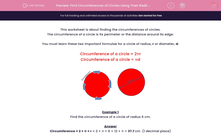# Find Circumferences of Circles Using Their Radii (1)

In this worksheet, students use formulae to calculate the circumferences of circles given their radii.This content is premium and exclusive to EdPlace subscribers.Key stage:  KS 2

Curriculum topic:   Maths and Numerical Reasoning

Curriculum subtopic:   2D Shapes: Circles, Angles and Bearings

Difficulty level:#### Worksheet Overview

This worksheet is about finding the circumferences of circles.

The circumference of a circle is its perimeter or the distance around its edge.

You must learn these two important formulae for a circle of radius, r or diameter, d:

Circumference of a circle = 2πr

Circumference of a circle = πdExample 1

Find the circumference of a circle of radius 6 cm.

Circumference = 2 × π × r = 2 × π × 6 = 12 × π = 37.7 cm  (1 decimal place)

Example 2

Find the circumference of a circle of diameter 6 cm.

Circumference = π × d = π × 6 = 18.8 cm  (1 decimal place)

Use a scientific calculator to work out your answer.  If you don't have one in the house, you can access one online.

### What is EdPlace?

We're your National Curriculum aligned online education content provider helping each child succeed in English, maths and science from year 1 to GCSE. With an EdPlace account you’ll be able to track and measure progress, helping each child achieve their best. We build confidence and attainment by personalising each child’s learning at a level that suits them.

Get started